# July 31, 2015

## Moving Average vs Windows Average

### Math, Programming, Moving Average and Standard Deviation

Averages are used in software all the time as a low-pass filter. One common filter method is a windowed average. This is an average using only a specific number of samples. Each new sample replaces the oldest sample, and the average taken across this set of data. Consider a scenario where a new point of data comes in every second, and you need to keep track of the average of this sample for the past 5 seconds.

 Data Average 1004 1003 1003 998 995 1000.6 993 998.4 1001 998 1008 999 1000 999.4 998 1000

Here we see 10 samples. The average doesn't start until the 5th sample because we want an average of 5 samples. After the 5th sample, the average is just an average of the previous 5 samples. To implement this, typically a circular buffer is used. We keep 5 samples, and always overwrite the oldest sample when a new sample arrives. Here are the 10 iterations of a circular buffer from the scenario above:

 1 2 3 4 5 6 7 8 9 10 1 1004 1004 1004 1004 1004 993 993 993 993 993 2 1003 1003 1003 1003 1003 1001 1001 1001 1001 3 1003 1003 1003 1003 1003 1008 1008 1008 4 998 998 998 998 998 1000 1000 5 995 995 995 995 995 998 Average 1000.6 998.4 998 999 999.4 1000

The new data is in bold. Notice how in column 6, the first row has been replaced. On the 7th column, the second row replaced, and so on. To implement this system in software is very simple, and there are some tricks you can do to keep the average up-to-date without having to sum the entire array each time which I have written about.

A windowed average suffers from a couple of drawbacks however. The biggest is the need to keep an array of all the previous samples. In a small processor one might not have enough memory to keep the array needed for a windowed average, especially if there are a lot of samples.

When doing filtering this can be solved instead using a first-order low-pass filter, known as a moving average. In the past I've written about using a software low-pass filter. I most often use this filter when reading what should, for the most part, be a DC value from an Analog to Digital Converter (ADC). Noise in this signal can be introduced for a number of reasons, but this simple filter is a very inexpensive way to take the noise out.

Here is the function for a first-order low-pass filter:

On = C In + ( 1 – C ) On-1

Where I is an array if input data, O is the output, and C is the filter coefficient with a value between 0 and 1. In implementation it is easier to think of this low-pass filter a poor-mans windowed average. Rather than think of a filter coefficient, consider the number of samples that will be averaged together. In this scenario, the equation becomes:

On = In + On-1 - On-1 / N

Where N is the number of samples. This acts like a windowed average over N samples—sort of. The difference is most apparent when the input makes a sudden change. Take for instance this setup: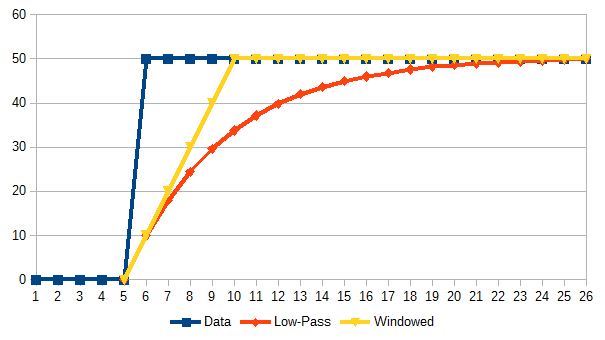Here is an average of the last 5 samples using both the windowed and low-pass methods. After 5 samples, the data makes a large change. The windowed average goes to the new values after 5 samples, where the low-pass filter takes much longer. Theoretically, the low-pass filter will never reach the new value as the new value will become an asymptote. If using integer numbers, it takes about 20 samples for this scenario. The larger the change, the longer it takes for the low-pass to reach this new value. When doing a software filter, this is not typically a problem—just something that must be accounted for when selecting the value for N.

One nice thing about using a moving average with N rather than a coefficient is the ability to avoid taxing operations like multiplies and divisions. If N is a power-of-two, the divide becomes a right-shift. This allows for a heavy filter coefficient, like 256, with barely any overhead. Here are the two lines of code needed to add a new sample to this average:

averageSum -= averageSum >> AVERAGE_SHIFT;
averageSum += newSample;

When the average is desired, it is simple the average sum divided by the number of coefficients.

average = averageSum >> AVERAGE_SHIFT;

Getting this average started often involves forcing the average sum to reflect the first sample. That is, force the average to be whatever the first sample is.

averageSum = firstSample << AVERAGE_SHIFT;

Since the low-pass filter takes time to react to changes, this allows the filter to start much closer to the actual average—assuming the first sample is fairly close to the average.

In addition to considerations about how much filtering is being done, the other factor is integer size. For example, if one wants a filter on 10-bit ADC data, if the average sum is 16-bits, then the value for the shift cannot be larger than 6, or N=64, without overflowing the sum.

The biggest benefit of using a moving average filter is that it only requires storage for an accumulator no matter how many samples are to be averaged together. Together with using a shift for a divide, it is both small and fast. This makes it very useful in small microprocessors, and high-speed digital signal processing.

There are times when a windowed average is better than a moving average. They work better in situations when the average value can change abruptly to a new steady state because they can react faster to the change. However, there is often a way to account for this using a moving average as well. Sometimes an abrupt change can be anticipated, and the average reset when it takes place. For instance, if monitoring a voltage that can be switched from a low level to higher level, the program might know this change was going to take place (perhaps because the software initiated it). When it happens, the moving average sum is reset with the first-sample method after the change has taken place. This allows the filter to react quickly to the change. This also works with the windowed average.

I personally use a windowed average when I have plenty of memory to work with if for no other reason than calculating the coefficient in meaningful terms is easy. For instance if one has 1 sample/sec, and averages 10 samples together, the filter is a 10 second average. With a moving average this is harder to quantify like this. Keeping a windowed average also lets one keep additional statistics such as standard deviation, which we will address in the next article. When memory and/or speed are key, nothing beats a power-of-two based moving average.

# August 01, 2015

## Windowed Standard Deviation

### Math, Programming, Moving Average and Standard Deviation

I wrote previously about how a windowed average can be used to do filtering, and made mention that a windowed average also allows for the calculation of standard deviation. In this article, I will address some acceleration for calculating standard deviation.

The first question one might ask is what having standard deviation is good for when filtering data. Standard deviation in a filter is a reflection of how much noise there is on the signal being filtered. The higher the standard deviation, the less signal to noise ratio. Knowing standard deviation helps select a filter window size so the signal can be extracted from the noisy data. That can often be calculated without having standard deviation in the finial code. But an adaptive filter might use standard deviation to determine the filter window.

Standard deviation might be used to indicate the incoming data is becoming unusable. It might also be used to detect sudden changes in the value being filtered. If the average signal level changes abruptly, standard deviation will rise as the filter transitions from the old value to the new.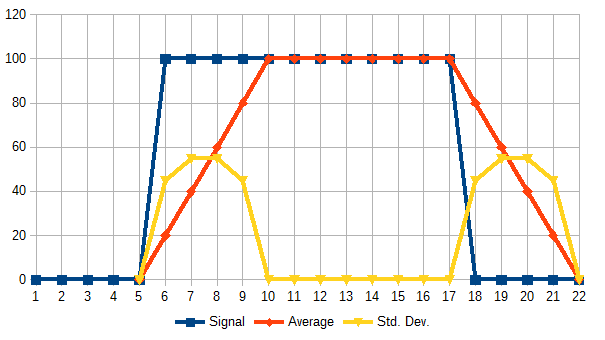Here we have a windowed average of 5 samples. The signal changes abruptly to 100, and then back to 0. The windowed average takes 5 samples to fully respond to this change. During that time, the standard deviation rises, and falls. In this way it can act as an indicator of such a change before the average reacts.

There are other uses for knowing standard deviation. By considering what standard deviation means about the data being acquired, additional uses can be conceived.

The classic equation for calculating standard deviation is as follows: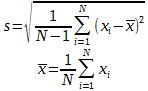Here x is an array of data points, N the number of data points, s is the corrected sample standard deviation—what most people simply call standard deviation, and x is the average of x. There is something one will notice about this calculation: the average must be known before standard deviation can be computed.

I've shown in previous articles a simple windowed average template class that can keep the average without having to do a complete calculation over the entire array. For standard deviation I didn't think this was possible because the average needed to be known before calculating standard deviation. Turns out I was mistaken. There is an iterative method. First, the iterative version of an average: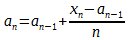Then standard deviation sum becomes: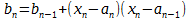And standard deviation at any point n is: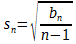That is nice for doing standard deviation in a single pass, but doesn't help us much with a continuous windowed standard deviation. In order to do this windowed, we need to be able to somehow subtract off older values from the running sum. This isn't possible here because of the divide by n-1 at the end.

Failing to find a solution to this, I went in search of an other method for doing windowed standard deviation and came across this article. The article presents this form for standard deviation: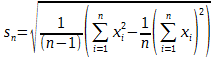This can be decomposed into two running sums: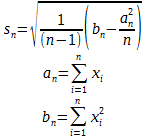Now that we have two running sums, we can make those circular.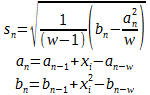Where w is the size of the window. We assume that an = 0 for all n < 0. That is, the circular buffers starts filled with zero. This has an other advantage in that the average is kept along side the standard deviation.

Here is a template class that does both a windowed average and standard deviation. It is derived from my earlier work that just did the average. As before, the template can do any type of input as long as it supports basic arithmetic functions, and has an overloaded version of the square root function sqrt.

#include <cmath> // <- For 'std::sqrt'.

//==============================================================================
// Template class for keeping a windowed average and standard deviation.
// Assumes math operations on 'TYPE' are computationally expensive, so used
// sparingly.
// For standard deviation to work, sqrt must be specialized to handle the
// template type.
//==============================================================================
templateclass TYPE >
class AverageStandardDeviation
{
protected:
// Storage of all the data points that make the average.
TYPE * array;

// A representation of zero in the type of this template.
TYPE const zero;

// Index into 'array' to save next data point.
unsigned currentIndex;

// How many data points are currently in array.
unsigned top;

// Length of 'array'.
unsigned length;

// Running sum used to compute average.
TYPE sum;

// Running sum of squares used for standard deviation.
TYPE squareSum;

public:
//------------------------------------------------------------------------
// Create moving average with 'lengthParameter' number of samples to be
// averaged together.
//------------------------------------------------------------------------
AverageStandardDeviation( unsigned lengthParameter )
:
zero( static_cast< TYPE >( 0 ) )
{
length = lengthParameter;

// Allocate storage for
array = new TYPE[ length ];

// We need a definition of zero, so make a cast to get this.
// NOTE: This is important if TYPE is truly a class.

reset();
}

//------------------------------------------------------------------------
// Reset all data in average.
// NOTE: Average result will be the equivalent of zero.
//------------------------------------------------------------------------
void reset()
{
// Empty array.
for ( currentIndex = 0; currentIndex < length; ++currentIndex )
array[ currentIndex ] = zero;

currentIndex = 0;
top = 0;
sum = zero;
squareSum = zero;
}

//------------------------------------------------------------------------
// Resize the number of samples used in average.
// If the size becomes larger, then the average will not change until
// If the size becomes smaller, then the last 'newLength' samples will be
// copied to new average.
//------------------------------------------------------------------------
void resize( unsigned newLength )
{
// Allocate memory for new array.
TYPE * newArray = new TYPE[ newLength ];

// Reset sums.
sum = zero;
squareSum = zero;

for ( unsigned count = newLength; count > 0--count )
{
unsigned index = count - 1;

// Do we have data to copy from the old array at this index?
if ( index < top )
{
// Get previous data index.
if ( currentIndex == 0 )
currentIndex = length - 1;
else
currentIndex -= 1;

// Save data in new array.
newArray[ index ] = array[ currentIndex ];

// Sum this data.
sum += newArray[ index ];
squareSum += newArray[ index ] * newArray[ index ];
}
else
// Set this position to zero.
newArray[ index ] = zero;
}

// Delete old array holder and switch to new array.
delete array;
array = newArray;

// Move top if the new length is lower than previous top.
if ( top > newLength )
top = newLength;

// New length.
length = newLength;

// Set new index location.
currentIndex = top;
if ( currentIndex >= length )
currentIndex = 0;
}

//------------------------------------------------------------------------
// Return the computed average.
// NOTE: Uses the data in the average thus far.  If average isn't full,
// then only those data points in the array are used.
//------------------------------------------------------------------------
TYPE getAverage() const
{
TYPE result;

if ( top > 0 )
result = sum / static_cast< TYPE >( top );
else
result = zero;

return result;
}

//------------------------------------------------------------------------
// Return the computed standard deviation.
//------------------------------------------------------------------------
TYPE getStandardDeviation() const
{
TYPE result;

if ( top > 1 )
{
using std::sqrt;
result  = sum * sum;
result /= static_cast< TYPE >( top );
result  = squareSum - result;
result /= static_cast< TYPE >( top - 1 );
result  = sqrt( result );
}
else
result = zero;

return result;
}

//------------------------------------------------------------------------
// Return the number of data points in the moving average so far.
//------------------------------------------------------------------------
unsigned getNumberOfDataPoints() const
{
return top;
}

//------------------------------------------------------------------------
// Add new data to average.
//------------------------------------------------------------------------
void push( TYPE newData )
{
TYPE oldPoint = array[ currentIndex ];

// Remove old data from average sum.
sum -= oldPoint;

// Remove old data from square sum.
squareSum -= oldPoint * oldPoint;

// Add new data to average sum.
sum += newData;

// Add new data to average square sum.
squareSum += newData * newData;

// Save new data.
array[ currentIndex ] = newData;

++currentIndex;

// If this marks the top...
if ( currentIndex > top )
// Update the top.
top = currentIndex;

// Wrap-around index.
if ( currentIndex >= length )
currentIndex = 0;
}

};

What's nice about this code is that adding a new sample to the average only requires a few operations. An array of the input data does need to be kept so it can be subtracted off, but both the average and standard deviation are kept as sums that can be quickly called upon for an exact result at any point. Standard deviation requires a few operations to translate the sums into a result, but this system is much faster than having to run through all the data points every time in order to get a result.

# August 02, 2015

## Moving Standard Deviation

### Math, Programming, Moving Average and Standard Deviation

In previous articles I wrote about the advantages of using a moving average over a windowed average, and how a continuous windowed standard deviation can be calculated. Keeping a continuous windowed average can be costly to memory. So the question is, can one keep a standard deviation when running the moving average algorithm?

The answer looks to be yes—mostly. The results of this standard deviation are close to the actual windowed standard deviation, but like the moving average filter itself, it is not an exact. Let us first explore the algorithm I implemented.

an = an-1 + (xnan-1) / w

bn = bn-1 + (xn – an-1)(xnan) – bn-1/w

sn= sqrt[ bn / ( w - 1 ) ]

Where a is is the moving average, b reflects the sum of variance, w is the window size, and s the standard deviation at anytime.

I wrote a simple program to generate Gaussian distributed random data with an initial standard deviation and ramping to a final standard deviation. Because the data is random, the actual standard deviation at any point over any number of samples can vary. However with enough samples and a windowed standard deviation there should be a clear trend of increasing standard deviation. I wanted to compare this estimated standard deviation method I created with windowed standard deviation to see how closely they follow one an other. To do this, I generated 1000 points of random data centered at an average of 0, and linearly increasing the standard deviation from 0 to 100.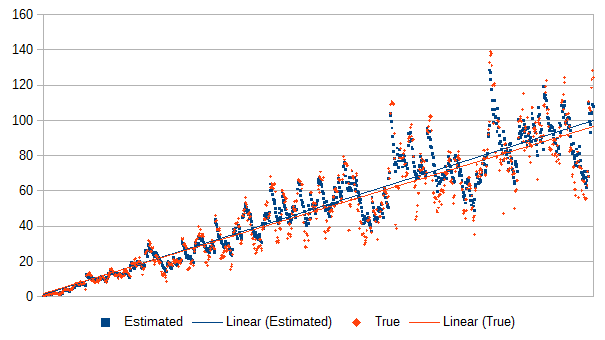In this plot we can see the moving standard deviation over a window of 10 samples vs the actual standard deviation over 10 samples. Included are the linearly regression lines for both data sets, showing a steady increase from a standard deviation of 0 to around 100. While the two methods are not exact, they tend to follow one an other. The true windowed standard deviation seems to be a little more erratic than the moving standard deviation. So the estimated seems to be dampened version of windowed standard deviation, and may prove useful to applications that require such things. This damping effect can be shown in a closer look at a set of data.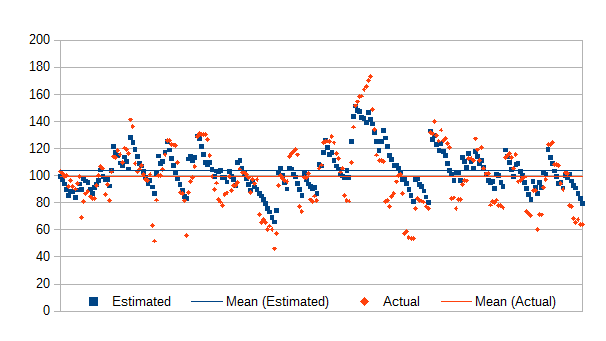This plot shows 256 standard deviation periods on a set of data. Notice that the moving standard deviation is on average closer to the overall standard deviation, hovering around 100. The windowed standard deviation for any window along the sample set can vary more wildly. However, the results are similar enough that the moving values should be a decent reflection of actual standard deviation.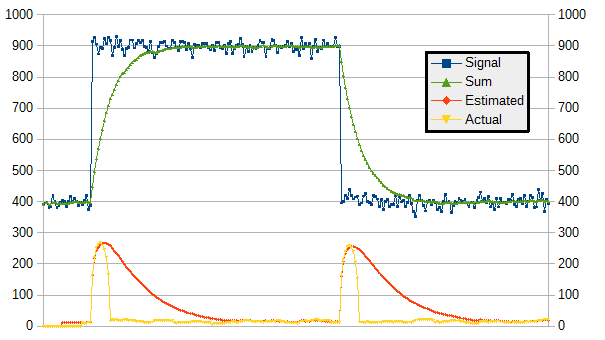This plot shows a rapid change in data, starting at 400, jumping to 900, then back to 400. The blue trace shows the incoming data, and the green the filtered data. In yellow is the true windowed standard deviation for the same time period as the filter. The orange shows the estimated standard deviation. While the standard deviation can ramp up quickly to meet the true deviation, it takes much longer to decay. What this says is the algorithm is quick to respond to increases in standard deviation, but slow for decreases.

Why this algorithm exhibits these characteristics is due to what the algorithm actually is: a squared error accumulator with a moving average drain. Error is accumulated just like normal standard deviation. However, there isn't a way to subtract off the exact amount of error at a latter time like that of a true windowed standard deviation. Instead, old error is removed with a low-pass filter just like that used in a moving average.

Software implementation requires two running values: an average, and a variance accumulator.

uint32_t varianceDrain = ( varianceSum / count );

int32_t lastAverage = average;
int32_t newAverage  = average;

newAverage += ( value - lastAverage ) / count;
average = newAverage;

varianceSum += ( value - lastAverage ) * ( value - newAverage );
varianceSum -= varianceDrain;

In this snip of code, the two running variables are average, and varianceSum. They are shown being updated with a new sample called value. The variable windowsSize might be a constant, and for speed can be converted into a power-of-2 so the divides can turn into shifts. The variable average can be used directly. Standard deviation requires one last conversion:

standardDeviation  = varianceSum;
standardDeviation /= ( count - 1 );
standardDeviation  = sqrt( standardDeviation );

The sqrt function is an integer square root, which takes up to the word bit size shift and addition operations to complete. They are much faster than the floating-point alternative. The square root can be eliminated if one can live with variance rather than standard deviation as a result.

To start the process, using a count variable rather than windowSize may be desired. Or the first sample could seed the average like this:

average = value * windowSize;

For implementation one must consider the worst-case values to avoid issues of overflow. To do this one must know the largest data value (xmax), the highest standard deviation (smax), and the word size (dmax). Note that if doing a saturated summation, only the largest meaningful values need to be considered. If standard deviation is being used as a threshold, then the maximum standard deviation can be the threshold as values above this are not necessary.

To avoid overflowing the average accumulator, insure w·xmax < dmax. For 10-bit data, and a window size of 64 (6-bits), 16-bits are needed for data accumulation which is the largest data type needed. Also be mindful of sine/unsigned. For a 16-bit unsigned integer dmax = 65535, but for a 16-bit signed dmax = 32767.

Variance is a square of error and grows more quickly than does the average. However one does not need to consider the data max (xmax)—only the highest standard deviation (smax). The restriction is that 2·w·smax < word size. So if the worst case standard deviation is 128 (7-bits) and the window size is 4096 (10-bits), the variance sum needs only to be 24-bits. Detecting variance saturation can be useful as well, which will indicate that the standard deviation is at or above it's maximum. If using the standard deviation for a threshold, this might actually be preferred because it means the accumulator only needs to cover up to the threshold but not actually account for anything above. On small microprocessors that allows smaller word sizes to be used, which can greatly improve speed.

//=============================================================================
// Name: movingAverage32.h
// Uses: Interface to compute a moving average and moving standard deviation.
// Date: 2015-08-02
// Author: Andrew Que (http://www.DrQue.net/)
// Revisions:
//   2015-08-02 - QUE - Creation.
//
// Copyright (c) 2015 Andrew Que (http://www.DrQue.net/)
// The MIT License (MIT).  See details at end.
//=============================================================================
#ifndef _MOVINGAVERAGE32_H_
#define _MOVINGAVERAGE32_H_

#include <stdint.h>

typedef struct Average32
{
int       count;       // Number of samples thus far (up to 'windowSize').
int       windowSize;  // Number of samples to average over.
int32_t   average;     // Current average (signed or unsigned).
uint32_t  varianceSum; // Running variance sum (always unsigned).
} Average32;

//-----------------------------------------------------------------------------
// USES:
//   Take the square root of a 32-bit integer.
// INPUT:
//   input - Value for which the root is to be taken.
// OUTPUT:
//   Integer square root of 'input'.
// NOTE:
//   The result of a taking the square root of a 32-bit number always fits
//   into a 16-bit number because sqrt( 2^32 ) = 2^(32/2) = 2^16.
//-----------------------------------------------------------------------------
static inline uint16_t sqrt_u32( uint32_t input )
{
uint32_t result = 0;

// The second-to-top bit is set.
uint32_t bit = UINT32_C( 1 ) << ( sizeof( uint32_t) * 8 - 2 );

// "bit" starts at the highest power of four <= the argument.
while ( bit > input )
bit >>= 2;

while ( bit != 0 )
{
if ( input >= result + bit )
{
input -= result + bit;
result = ( result >> 1 ) + bit;
}
else
result >>= 1;

bit >>= 2;
}

return (uint16_t)result;
}

//-----------------------------------------------------------------------------
// USES:
//   Setup 32-bit moving average.
// INPUT:
//   average - 32-bit moving average instance.
//   windowsSize - Number of samples to average over.
//   shift - Shift to scale input to add precision.  Optional.
// OUTPUT:
//   Modified 'average'.
//   Nothing is returned.
//-----------------------------------------------------------------------------
static inline void average32_Init
(
Average32 * average,
int32_t windowSize
)
{
average->windowSize  = windowSize;
average->count       = 0;
average->average     = 0;
average->varianceSum = 0;
}

//-----------------------------------------------------------------------------
// USES:
//   Add a data point to the moving average.
// INPUT:
//   average - 32-bit moving average instance.
//   value - New value to incorporate.
// OUTPUT:
//   Modified 'average'.
//   Nothing is returned.
//-----------------------------------------------------------------------------
static inline void average32_AddValue( Average32 * average, int32_t value )
{
int maxCount = ( 1 << average->windowSize );

int32_t  lastAverage = average->average;
int32_t  newAverage  = average->average;

// Roll-off old data.
newAverage -= ( lastAverage >> average->windowSize );

// Accumulate new data.
newAverage += value;

// Use the very first sample as a representation of all data to come.
// (Optional--only needed if desiring accurate results in start up.)
if ( 0 == average->count )
{
// Make the first value the starting average.
newAverage <<= average->windowSize;

// Set last is the same as first to force variance at 0 for first sample.
lastAverage = newAverage;
}
else
// A change to the most-significant bit means the accumulator has overflowed.
// (Optional if average saturation is needed.)
if ( ( newAverage ^ average->average ) >> ( sizeof( newAverage ) * 8 - 1 ) )
{
// Saturate negative or positive?
if ( average->average < 0 )
newAverage = INT32_MIN;
else
newAverage = INT32_MAX;
}

// Save new average.
average->average = newAverage;

// Calculate variance the new sample adds.
uint32_t variance =
( value - ( newAverage  >> average->windowSize ) )
* ( value - ( lastAverage >> average->windowSize ) );

// Roll-off old variance.
// (Optional if.  Can be removed if always waiting for at least
//  average->windowSize samples before calculating result.)
if ( average->count >= maxCount )
average->varianceSum -= ( average->varianceSum >> average->windowSize );

// Accumulate new variance.
variance += average->varianceSum;

// Unsigned saturate test and correction.
// (Optional if saturation is needed.)
variance |= -( variance < average->varianceSum );

average->varianceSum = variance;

// Keep incrementing count if just getting started.
// (Optional--only needed if desiring accurate results in start up.)
if ( average->count < maxCount )
average->count += 1;
}

//-----------------------------------------------------------------------------
// USES:
//   Return moving average of data up to this point.
// INPUT:
//   average - 32-bit moving average instance.
// OUTPUT:
//   The moving average of all data.
//-----------------------------------------------------------------------------
static inline int32_t average32_Get( Average32 const * average )
{
return average->average >> average->windowSize;
}

//-----------------------------------------------------------------------------
// USES:
//   Return approximate standard deviation of data up to this point.
// INPUT:
//   average - 32-bit moving average instance.
// OUTPUT:
//   An approximation of standard deviation of data.
//-----------------------------------------------------------------------------
static inline uint32_t average32_GetStandardDeviation
(
Average32 const * average
)
{
uint32_t standardDeviation = average->varianceSum;

// Avoid a divide-by-zero.
// (Can be removed if using shifts instead.)
if ( average->count > 1 )
{
// Division can be replaced with shift, but the result will be biased.
// Since the standard deviation is an estimate anyway, that shouldn't be
// a problem.
standardDeviation /= ( average->count - 1 );
//standardDeviation >>= average->windowSize;  // Divide by shift.

// The square root can be skipped, which results in variance rather than
// standard deviation.
standardDeviation = sqrt_u32( standardDeviation );
}

return standardDeviation;
}

#endif // _MOVINGAVERAGE32_H_

//
// Copyright (c) 2015 Andrew Que (http://www.DrQue.net/)
//
// Permission is hereby granted, free of charge, to any person obtaining a copy
// of this software and associated documentation files (the "Software"), to deal
// in the Software without restriction, including without limitation the rights
// to use, copy, modify, merge, publish, distribute, sublicense, and/or sell
// copies of the Software, and to permit persons to whom the Software is
// furnished to do so, subject to the following conditions:
//
// The above copyright notice and this permission notice shall be included in
// all copies or substantial portions of the Software.
//
// THE SOFTWARE IS PROVIDED "AS IS", WITHOUT WARRANTY OF ANY KIND, EXPRESS OR
// IMPLIED, INCLUDING BUT NOT LIMITED TO THE WARRANTIES OF MERCHANTABILITY,
// FITNESS FOR A PARTICULAR PURPOSE AND NONINFRINGEMENT. IN NO EVENT SHALL THE
// AUTHORS OR COPYRIGHT HOLDERS BE LIABLE FOR ANY CLAIM, DAMAGES OR OTHER
// LIABILITY, WHETHER IN AN ACTION OF CONTRACT, TORT OR OTHERWISE, ARISING FROM,
// OUT OF OR IN CONNECTION WITH THE SOFTWARE OR THE USE OR OTHER DEALINGS IN
// THE SOFTWARE.
//

The above is a C implementation for 32-bit signed data with most features written in. It can be cut down depending on the need of the application. For instance, to make this system faster biased variance can be used rather than true standard deviation. This turns the divide into a shift, and removes the square root function. These changes can be made with ease:

• From signed to unsigned. This allows the average saturation code to be simplified to a single line just like it is for the unsigned saturation of the variance sum.

• No accumulator saturation. If you don't need it, this will save a couple instructions.

• No initial condition setting. Requires average to have at least a window size of samples before results are accurate.

• Biased standard deviation. Change the divide to a shift when calculating standard deviation and increase the speed. Almost always a good idea.

• Use variance instead of standard deviation. Just remove the square root and save that amount of time.

The code can easily be changed to other bit widths as well. To change to 16-bit code, replace all occurrences of 16 with 8, and all 32 with 16. The switch of 16 to 8 is for the result of the square root function which is moot because it's promoted when used anyway. In the same way, this code can also be scaled up to 64-bits.